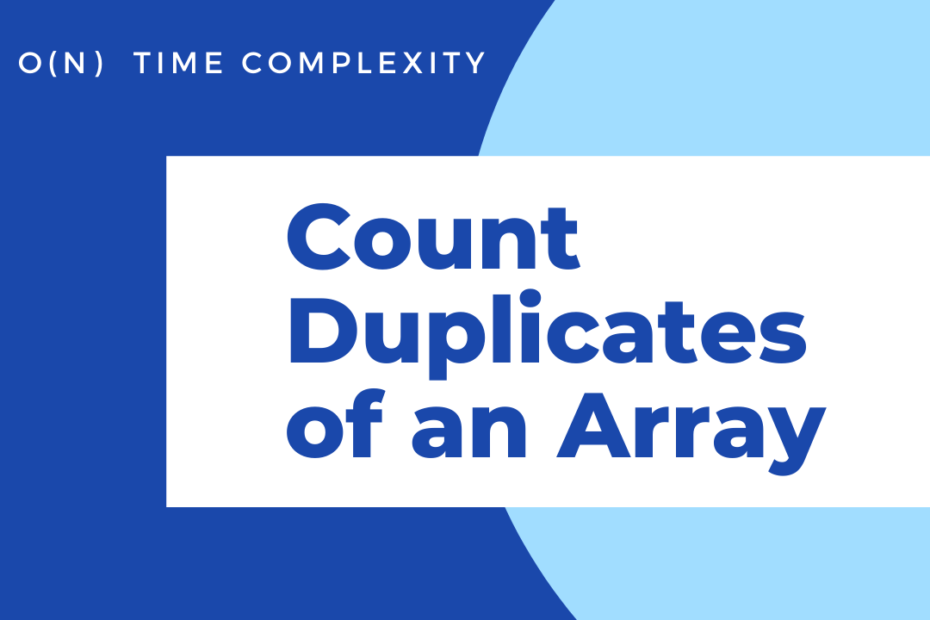# Count the Duplicates of Array O(N)

• DSA

In the given problem, we have a sorted array, and we need to count the duplicates of each element. In simple words, we should print the no.of times each element occurs.

## Example

``````Input: [1,2,2,4,5,5,5,6]
Output:
2 appears 2 times
5 appears 3 times``````

## Solution approach

Since we have a sorted array, things become much easier. Once we traverse through an element, if it has a duplicate then definitely the next element will be the same, and so on until we traverse through the last duplicate element of the same.

IF a[i] == a[i+1] THEN Duplicates++

With this method we can find

• No of repeating elements
• Duplicates of each element and their count

## Code to find Duplicates in an Array

``````#include<iostream>
using namespace std;
int main()
{
int a={1,4,6,6,8,11,11,15,15,15,16};
int j=0;
for(int i=0;i<11-1;i++)
{
if(a[i]==a[i+1])
{
j=i+1;
//j contains second duplicate element
while(a[j]==a[i])j++;
cout<<a[i]<<" appears "<<j-i<<" times "<<endl;
//skip all j's we covered.
i=j-1;
}
}
}
``````

## Output

``````6 appears 2 times
11 appears 2 times
15 appears 3 times``````

## Conclusion

I hope you found this helpful, always remember that whenever we\’ve got a sorted array, in most cases we can carry out any kind of operation in a single iteration with O(N) Time Complexity. This post is a part of my #30DaysChallenge to write a blog post every day on what I learn, Cheers~ Abhiram Reddy.